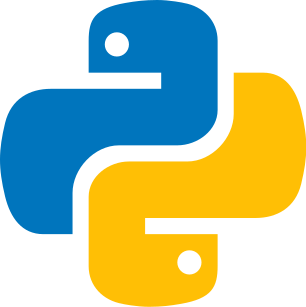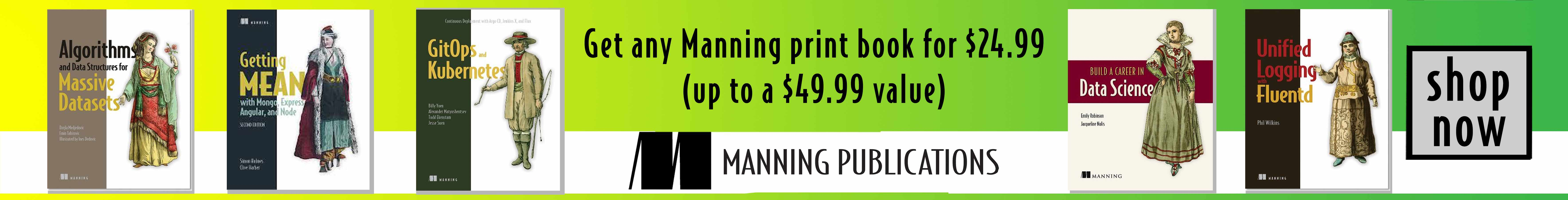Codistwa

# Learn Linear algebra

## Vectors with Numpy and Matplotlib

Last update : February 11th, 2022

Linear algebra studies the elements present in vector spaces that can perform linear transformations (displacement, enlargement, etc.). These transformations are done using operations (sum, multiplication).### What is a vector?

A vector has these two pieces of information:

• the direction (points of the vector)

A vector with two elements will have points (coordinates) corresponding to x and y. Thus, x will be the first point, y the second.

• the magnitude (length)

This is the total number of points. 2 for a vector in 2 dimensions.

A vector can be displayed as a column or a row.

In the first comic strip, the direction of a vector is represented by the red arrow going up and to the right. The character is walking in the direction of the black circle. The second comic panel represents the length of the vector with the black arrow below it. Finally, in the last comic panel, an example of vector use is demonstrated via a map and coordinates.

#### Codepython
1!pip install sympy as sym
2!pip install  matplotlib.pyplot as plt
3!pip install  numpy as np
4from IPython.display import display,Math
5
6scalar =  # scalar
7row = np.array([2,1], dtype=object) # row matrice / vector
8col = np.array([ , [-1] ], dtype=object) # column matrice / vector
9
10display(Math(sym.latex(sym.sympify(scalar))))
11display(Math(sym.latex(sym.sympify(row))))
12display(Math(sym.latex(sym.sympify(col))))
13
14ax = plt.axes()
15ax.arrow(0, 0, row, row, head_width=0.2, label='$row$', head_length=0.2, color='r', length_includes_head=True)
16ax.arrow(0, 0, col, col, head_width=0.2, label='$column$', head_length=0.2, color='b', length_includes_head=True)
17
18plt.axis('square')
19plt.legend()
20
21plt.grid()
22plt.show()
23
24plt.title('Row and columns vectors',fontsize=12)
25plt.savefig('row_col_vectors.png', bbox_inches='tight')

### What is a 3D vector?

A vector with three elements will have points corresponding to x, y, and z. Thus, x will be the first point, y the second, z the third. It is, therefore, a vector in 3 dimensions.

There are vectors with a lot of points, and the representation is uncompromising; that's why we limit ourselves here to a 3D model.

The room represents the space in 3D, and we see the floor and the walls. With the staircase, the character goes straight up; it is a scene located in a 3D space. Finally, in the last panel, the character walks towards a red cross which is the destination, with the GPS icon above this cross. The path is linear here, but in general, we do not walk straight. It is these complexities that allow the use of vectors.

#### Codepython
1vector1_3D = np.array([ , , [-2] ], dtype=object)
2vector2_3D = np.array([ , [-4],  ], dtype=object)
3vector3_3D = np.array([ , ,  ], dtype=object)
4
5display(Math(sym.latex(sym.sympify(vector1_3D))))
6display(Math(sym.latex(sym.sympify(vector2_3D))))
7display(Math(sym.latex(sym.sympify(vector3_3D))))
8
9fig = plt.figure()
11
12# draw vectors
13ax.plot([0,vector1_3D],[0,vector1_3D],[0,vector1_3D],'b',linewidth=3)
14ax.plot([0,vector2_3D],[0,vector2_3D],[0,vector2_3D],'r',linewidth=3)
15ax.plot([0,vector3_3D],[0,vector3_3D],[0,vector3_3D],'g',linewidth=3)
16
17# guidelines
18ax.plot([-5,5],[0,0],[0,0],'--',color=[.7,.7,.7])
19ax.plot([0,0],[-5,5],[0,0],'--',color=[.7,.7,.7])
20ax.plot([0,0],[0,0],[-5,5],'--',color=[.7,.7,.7])
21
22ax.set_xlim3d(-5,5)
23ax.set_ylim3d(-5,5)
24ax.set_zlim3d(-5,5)
25
26ax.set_xlabel('X')
27ax.set_ylabel('Y')
28ax.set_zlabel('Z')
29
30fig.savefig('3d_vectors.png', bbox_inches='tight')
31
32plt.show()

### Sum of two vectors

The sum is the simplest operation. We will add the vectors according to the position of their points (coordinates). The first point of the first vector adds up to the first point of the second vector. In the end, we obtain a new vector. This is called the Chasles relationship.

Graphically it corresponds to a new vector that connects the two others.

The sum of two vectors is defined graphically as follows: we put the two vectors end to end so that the endpoint of the first vector coincides with the beginning point of the second vector. The third vector connects the initial point of the first vector to the terminal point of the second vector. We can check this with the arrows.

In these comic strips, the combination of vectors is represented with a character who will go to two destinations. We could consider that he has a destination but that he makes a stop. And the path between the departure and the arrival is the sum of these two steps.

#### Codepython
1vector1 = np.array([ , [-2] ], dtype=object)
2vector2 = np.array([ , [-4] ], dtype=object)
3
4display(Math(sym.latex(sym.sympify(vector1))))
5display(Math(sym.latex(sym.sympify(vector2))))
6
7vector_sum = vector1 + vector2
9
10vector1_show = sym.latex(sym.sympify(vector1))
11vector2_show = sym.latex(sym.sympify(vector2))
12vector3_show = sym.latex(sym.sympify(vector_sum))
13
14display(Math('%s + %s=%s' %(vector1_show, vector2_show, vector3_show)))
15
16ax = plt.axes()
17ax.arrow(0, 0, vector1, vector1, label='$vector1$', head_width=0.2, head_length=0.2, color='b', length_includes_head=True)
18ax.arrow(vector1, vector1, vector2, vector2,  label='$vector2$', head_width=0.2, head_length=0.2, color='r', length_includes_head=True)
19ax.arrow(0, 0, vector_sum, vector_sum, label='$vector1 + vector2$', head_width=0.2, head_length=0.2, color='g', length_includes_head=True)
20
21plt.axis('on')
22plt.legend()
23plt.grid()
24
25plt.title('Sum of two vectors',fontsize=12)
26plt.savefig('sum_two_vectors.png', bbox_inches='tight')
27
28plt.show()

### Difference of two vectors

We will subtract the vectors according to the position of their points. The first point of the first vector is subtracted from the first point of the second vector. In the end, we obtain a new vector. You can also add by inverting the points of the second vector; add a - to each point. This trick helps to represent the result graphically.

Graphically this also corresponds to a new vector that connects the other two.

Here the difference is represented by the character who goes back and forth. And it is, therefore, the combination of these two steps that constitute the difference.

#### Codepython
1vector1 = np.array([ , [-2] ], dtype=object)
2vector2 = np.array([ , [-4] ], dtype=object)
3
4vector2_inverse = np.array([ [-2],  ], dtype=object)
5
6vector_difference = vector1 - vector2
7vector4_show = sym.latex(sym.sympify(vector_difference))
8
9display(Math('%s - %s=%s' %(vector1_show, vector2_show, vector4_show)))
10
11ax = plt.axes()
12ax.arrow(0, 0, vector1, vector1, head_width=0.2, label='$vector1$', head_length=0.2, color='b', length_includes_head=True)
13ax.arrow(vector1, vector1, vector2_inverse, vector2_inverse, label='$vector2$', head_width=0.2, head_length=0.2, color='r', length_includes_head=True)
14ax.arrow(0, 0, vector_difference, vector_difference, label='$vector1-vector2$', head_width=0.2, head_length=0.2, color='g', length_includes_head=True)
15
16plt.title('Difference of two vectors',fontsize=12)
17
18plt.axis('on')
19plt.legend()
20
21plt.grid()
22plt.show()
23
24plt.savefig('difference_two_vectors.png', bbox_inches='tight')

### Multiplying a vector by a scalar

We can multiply a vector by a scalar. The scalar is a single number. If we multiply it by 2 we will double it. The scalar will multiply all the points of the vector.

The character has to get to a destination in these comic strips, but he can't continue on foot. He's halfway there. He can finish the other half with the help of a bicycle; the bicycle will allow him to arrive in time quickly; it is a kind of gas pedal.

#### Codepython
1vector_scalar = vector1 * scalar
2vector5_show = sym.latex(sym.sympify(vector_scalar))
3
4display(Math('%s X % s=%s' %(vector1_show, scalar, vector5_show)))

### Multiplying a vector by a vector - the dot product

The dot product is two vectors whose result is a scalar (unique number). Thus, the result is a single number, unlike the vector product. You can do this calculation with Numpy or Python with the @ operation.

In these comic strips, the dot product is illustrated with the boost. Indeed, the multiplication of several vectors creates a force. Therefore, we can consider the wind allowing the character to go faster as multiplied vectors whose result is a greater speed.

#### Codepython
1vector_dot = vector1 * vector2
2
3v1 = np.array([1, 2, 3])
4v2 = np.array([-3, 1, -4])
5
6print(v1 @ v2) # python operator https://www.python.org/dev/peps/pep-0465/
7print(np.dot(v1, v2))

#### FormulaWith vectors, we can study linear transformations by performing additions, subtractions and products.